# PSAT Math : How to find the length of the diagonal of a rectangle

## Example Questions

### Example Question #2 : How To Find The Length Of The Diagonal Of A Rectangle

What is the length of the diagonal of a rectangle that is 3 feet long and 4 feet wide?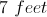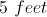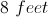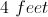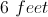Explanation:

The diagonal of the rectangle is equivalent to finding the length of the hypotenuse of a right triangle with sides 3 and 4. Using the Pythagorean Theorem: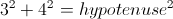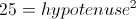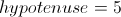Therefore the diagonal of the rectangle is 5 feet.

### Example Question #3 : How To Find The Length Of The Diagonal Of A Rectangle

The length and width of a rectangle are in the ratio of 3:4. If the rectangle has an area of 108 square centimeters, what is the length of the diagonal?

12 centimeters

15 centimeters

9 centimeters

24 centimeters

18 centimeters

15 centimeters

Explanation:

The length and width of the rectangle are in a ratio of 3:4, so the sides can be written as 3x and 4x.

We also know the area, so we write an equation and solve for x:

(3x)(4x) = 12x= 108.

x2 = 9

x = 3

Now we can recalculate the length and the width:

length = 3x = 3(3) = 9 centimeters

width = 4x = 4(3) = 12 centimeters

Using the Pythagorean Theorem we can find the diagonal, c:

length2 + width2 = c2

92 + 12= c2

81 + 144 = c2

225 = c2

= 15 centimeters